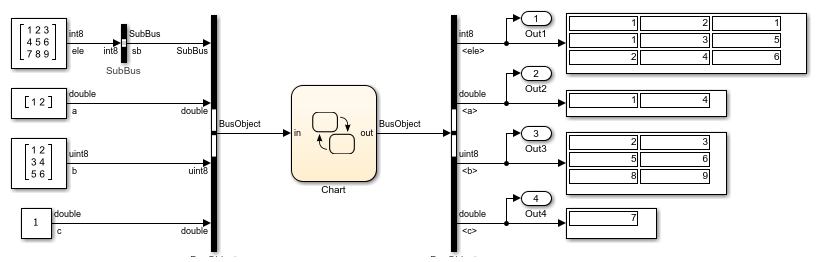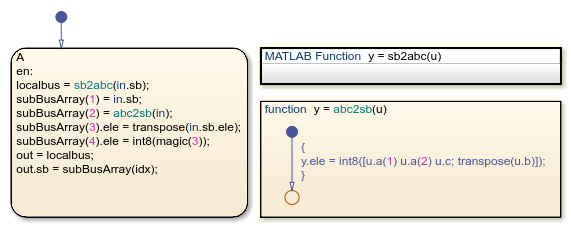# Connect Structures in MATLAB Functions to Simulink Bus Signals

This example shows how to use MATLAB® structures in a Stateflow® chart. MATLAB structures enable you to bundle data of different sizes and types together into a single variable in a MATLAB function. You can create a MATLAB structure to:

• Store related data in a local or persistent variable of a MATLAB function

• Read from or write to a local Stateflow structure

• Interface with a Simulink® bus signal at an input or output port

### Define Structures in MATLAB Functions

In this example, a Stateflow chart processes data from one Simulink bus signal and outputs the result to another Simulink bus signal. Both the input and output bus signals are defined by the `Simulink.Bus` (Simulink) object `BusObject`. These buses have four fields: `sb`, `a`, `b`, and `c`. The field `sb` is also a bus signal defined by the `Simulink.Bus` object `SubBus`. It has one field called `ele`.In the chart, the Simulink bus signals interface with the Stateflow structures `in` and `out`. The functions `abc2sb` and `sb2abc` extract information from the input structure and store it in the local Stateflow structures `localbus` and `subbus`. Then, the chart writes to the output structure by combining the values of these local structures. For more information on Stateflow structures, see Access Bus Signals Through Stateflow Structures.The MATLAB® function `sb2abc` takes a Stateflow structure of type `SubBus` and returns a Stateflow structure of type `BusObject`. The function decomposes the value of the field `ele` from its input into three components: a vector, a 3-by-2 matrix, and a scalar. The function stores these components in the local MATLAB `struct` `Y` that has the same structure as the `Simulink.Bus` object `BusObject`. Then, the function assigns the value of the MATLAB `struct` `Y` to the output structure `y`.

```function y = sb2abc(u) ```
```% extract data from input structure ```
```E = int8(magic(3)); A = double(u.ele(1:2,1)); B = uint8(u.ele(:,2:3)); C = double(u.ele(3,1)); ```
```% create local structure ```
```X = struct('ele',E); Y = struct('sb',X,'a',A,'b',B,'c',C); ```
```% assign value to output structure ```
```y = Y; end ```

### Define Input and Output Structures

In a MATLAB function, access a local Stateflow structure or interface with a Simulink bus signal by defining input and output structures for the function:

1. In the base workspace, create a `Simulink.Bus` object that defines the structure data type.

2. In the Symbols pane, select the function input or output.

3. In the Property Inspector, set the Type property to `Bus: <object name>`. Replace <object name> with the name of the `Simulink.Bus` object that defines the Stateflow structure.

For example, in the function `sb2abc`:

• The Type property of the input structure `u` is specified as `Bus: SubBus`.

• The Type property of the output structure `y` is specified as `Bus: BusObject`.

### Define Local and Persistent Structure Variables

To store related data in a single variable inside a MATLAB function, you can create a MATLAB `struct` as a local or persistent variable. For example, the function `sb2abc` defines two local MATLAB structures to temporarily store the data extracted from the input structure `u` before writing to the output structure `y`:

• `X` is a scalar `struct` with a single field called `ele`. This field contains a 3-by-3 matrix of type `int8`, which matches the structure of the `Simulink.Bus` object `SubBus`.

• `Y` is a scalar `struct` with four fields: `sb` is a substructure of type `SubBus`, `a` is a two-dimensional vector of type `double`, `b` is a 3-by-2 matrix of type `uint8`, and `c` is a scalar of type `double`. These fields match the structure of the `Simulink.Bus` object `BusObject`.